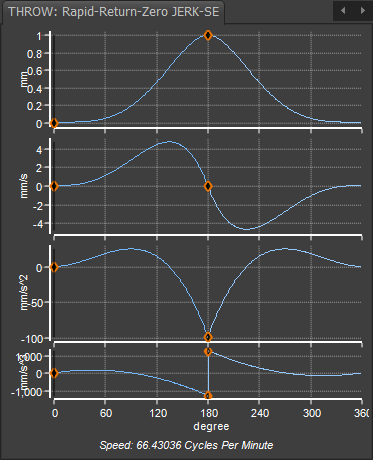﻿ MotionDesigner Reference & User Interface > Motion Laws / Cam-Laws for Segments > Throw Motion-Law > Throw Rapid-Return 2: Zero-Jerk @ Start/End Motion-Law, Finite at Mid-Point

# Throw Rapid-Return 2: Zero-Jerk @ Start/End Motion-Law, Finite at Mid-Point

### Throw: Rapid-Return with Zero Jerk

#### The Throw Motion-Law

Unlike other motion-laws, the Throw motion-law rises from its Start-Position to its maximum displacement, and then returns to its Start-Position.

To give you maximum flexibility, use two Flexible Polynomial segments to design the Throw motion-law. Usually, the two segments have equal durations, but it is not necessary.

#### Throw: Rapid-Return 2 - Zero Jerk at Start and End, unspecified at its mid-point.

Because you use two Flexible-Polynomial segments to design the Throw motion-law, you can enter different motion-values and motion-constraints at its start, its maximum displacement, and at its end.

All Throw motion-laws have zero velocity and acceleration at their start and end.

This Throw motion-law also has zero Jerk at its start and end.

This Throw has a Quick-Return because it has a LARGE negative acceleration, and unspecified Jerk, at its maximum displacement - the transition from Rise to Return.

The Throw type motion-law it typically symmetrical. You can make it asymmetrical, if you move the X-axis value at its mid-point.

#### Two Segment 'Rapid-Return with Zero Jerk at Start/End,  2'Motion-Values of each motion-derivative

Start of rise segment 1 :

Position = 0

Velocity = 0

Acceleration = 0

Jerk = 0

Mid-Point :

Position = 1

Velocity = 0

Acceleration = Unspecified (Actual value = -98.mm/s/s )

Jerk = Unspecified (Actual value = ±1303mm/s/s/s )

End of return Segment 2 :

Position 2 = 0

Velocity = 0

Acceleration = 0

Jerk = 0